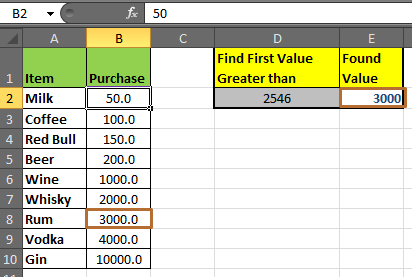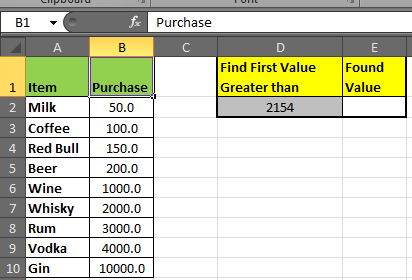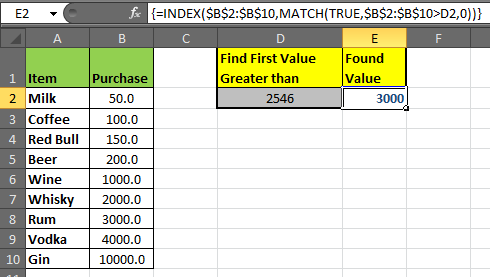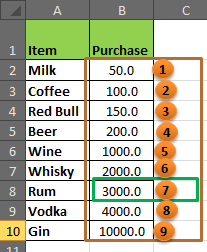# Retrieving the first value in a list that is greater / smaller than a specified valueThe generic formula for finding the first number from a list that is greater than a given number is.

 =INDEX(list,match(TRUE,list>number,0))

Example:

Here, I have created a list of goods and their prices. The price list is in range B2:B10. Now we want to find the first Price that is greater than 2154 ( a random number) in cell E2.Enter this Formula in cell E2 and hit CTRL+SHIFT+ENTER (**necessary to convert your formula into array formula) simultaneously:

 {=INDEX(\$B\$2:\$B\$10,MATCH(TRUE,\$B\$2:\$B\$10>D2,0))}We got our answer. It's 3000. Now, whenever you change the value in D2 you will have a changed value in E2 (of course if matches to other criteria).

Explanation:

Every Formula works inside out. Most of the inner functions are compiled first and then outer.So let’s take a look at each segment of the formula.MATCH(TRUE,\$B\$2:\$B\$10>D2,0)

Match function returns the index of a value in a list.

The basic syntax of Match is:

MATCH(lookup_value,lookup_array,[match_type])

1. In our case, the lookup_value is TRUE. (why?) and

2. Lookup_array is ,\$B\$2:\$B\$10>D2. This will return an array of boolean values where the condition is matched. If you select this section and press the f9 key, you will see this.

{FALSE;FALSE;FALSE;FALSE;FALSE;FALSE;TRUE;TRUE;TRUE}. That is why our lookup value is ‘TRUE’. It will return the index number of first true value and that is 7.

3. Match_Type is 0, 0 is for an exact match.

INDEX(\$B\$2:\$B\$10(TRUE,\$B\$2:\$B\$10>D2,0))

Index only returns a value from a list of given Index.

The basic syntax of Index is:

INDEX (array,row_number)

1. In our case, the array is \$B\$2:\$B\$10. This is the list from which we want to get our results.
2. Row_number is evaluated using MATCH(TRUE,\$B\$2:\$B\$10>D2,0). Which gives us 7.Hence the final match gives index row number 7. And using that INDEX returns the value at index 7.

Similarly, if you want to find the first number in a list that is less than the given value, just replace ‘<’ with ‘>’ in the formula.

## Formula to find the first number in a list that is less than the given value

Just change the logical operator to < from >. It's done. You have the first smallest number in the list.

 {=INDEX(\$B\$2:\$B\$10,MATCH(TRUE,\$B\$2:\$B\$10

Now you know how to find the first larger/smaller value in a list. I hope it was resourceful for you. Use the comment section if it wasn’t and post your query.

Related Articles:

How to Vlookup Top 5 Values with Duplicate Values Using INDEX-MATCH in Excel

How to Retrieve Latest Price in Excel

How to Get Last Value In Column in Excel

How to Get Position of First Partial Match in Excel

Popular Articles:

50 Excel Shortcuts to Increase Your Productivity

How to use the VLOOKUP Function in Excel

How to use the COUNTIF function in Excel

How to use the SUMIF Function in Excel

1.Now, what if you wanted to return the item that matches the number you found. For example, in the first example, I'd want to find the item rum because it is the first item that costs more than 2546. So I want to find the item that costs more than a certain amount. How would I do that?

•Hi Paige,

It is simple, just give the reference of the items for INDEX instead of values. For example we have =INDEX(list,match(TRUE,list>number,0)). Change it to, =INDEX(item_list,match(TRUE,list>number,0))

See, here I have change the list to item_list (the list of items, not prices) for INDEX only (not for match). Now it will return item instead of price.

I hope it works for you.

•This last formula is exactly what I want but don't quite understand the item_list.
I have a number in column A if lower than H14 I want to return the value from column B in in that same row.

2.Hi sir,
the given formula is most helpful to find first Grater Value.

I use this formula to find first small number; but i cant find first small value. It return every time smallest value..

can you help me.

•Hi Dilip,

3.Vytautas Gabriunas

Merely changing the logical operator to "" is not enough. This just retrieves always the first number in the list. In order to make it work the numbers in the list must be rearranged in descending order.
Nevertheless, it is an extremely useful formula indeed. Thanks !!!!!

4.David Atherton

No use if data is not sorted into order first. I am trying to find how many days from a given date/price that the price has gone up (made a new high). Prices go up/down every day. All these Excel functions seems to need the data in order.

5.What if I want to retrieve the value of the cell BEFORE the first number greater than the specified value?

•You can adjust the index reference: for example, if the greatest value you are finding is in D3 of range D2:D7 you wand value from B3, then give range of B2:B7:
=INDEX(B2:B7,MATCH(TRUE,D2:D7>12,0))

If you want value from the above cell of the greatest cell then adjust the index range to one cell above from that range. for example, if the greatest value is in D3 of range D2:D7 then give index as D1:D6:
=INDEX(D1:D6,MATCH(TRUE,D2:D7>12,0))

Remember to enter the formula as an array formula. (CTRL+SHIFT+ENTER)

6.Dear All,

Really amazing
Chirag

7.Dear All

Really amazing tips this is
Regards,
CHirag

8.hello. Can you please help me on this one? i need to have a formula that will pick the nearest number that is less than a number and get it from row 1a - 4b.
for example, that value is 3000 and choose 2a, the answer should be 2500

1a - 1.00 - 417.00
2a 50.00 1.00 2,083.00 2,500.00
1b 75.00 1.00 3,125.00 3,542.00
2b 100.00 1.00 4,167.00 4,583.00
3b 125.00 1.00 5,208.00 5,625.00
4b 150.00 1.00 6,250.00 6,667.00

Hope to get a response. thank you so much in advance!!

9.Thank you for posting this. How do you write the function if you want to find the first instance bottom-up instead of top-down? The examples above only seem to be top-down in the array.

Thanks!

•To be clear, this function is the one I want to use, but for it to read bottom-up. Thank you.

•have you ever found answers on your questions? this is what I am looking for too.

10.Unless I'm mistaken (entirely possible):
Does the formula find the number *just below* the criteria number or does it in fact find the *lowest* number in the column?

EX: Criteria=81
Greater Than=95
Smaller Than=54

Shouldn't "Smaller Than" be 70?

Thanks for providing these formulae and answer !

11.I have to create a sheet showing a sales reps revenue by client over a 15 month period with out showing the clients with out any revenue. I have no idea how to do this. Please help.

•Hi Joe,

You can simply create a snapshot and can add revenue for those clients who have given you revenue. For more info, we recommend you to login on www.excelforum.com and post your query there to get instant and clear solution for your query.

Thanks,

12.Very useful - thank you 🙂

13.I really need this formula..Thanks.

14.I have been searching for this formula for 3 weeks!!! Fantastic

15.Hi,
To all of you I really want to know how to merge csv file without using copy paste?

•Hi Shyamdhar,

16.